# Differential Calculus Notes - JEE

## JEE: Differential Calculus Notes - JEE

The document Differential Calculus Notes - JEE is a part of JEE category.
All you need of JEE at this link: JEE
`Differential Calculus is one of the most important topics in the preparation of  IIT JEE. This is the easiest part of Calculus and there is no doubt in the fact that it is scoring too. It is also important to attain proficiency in Differential Calculus as it is a prerequisite to the learning of Integral Calculus too.Differential Calculus is a branch of mathematical analysis which deals with the problem of finding the rate of change of a function with respect to the variable on which it depends. So, differential calculus is basically concerned with the calculation of derivatives for using them in problems involving non constant rates of change. Applications also include computation of maximum and minimum values of a function.  The study of Differential Calculus includes Functions, Sets and Relations though they are considered to be a part of Algebra. Limits, Continuity and Differentiability are the favorite topics of those who have a bent towards Differential Calculus. It is not only an easy topic but also fetches direct question in the examination. A person who has already done a good practice of this chapter is also likely to do well in the next topic of Differentiation. The lifeline of Differential Calculus is basically the topics which include the application of Derivatives i.e.Tangent and Normal and Maxima and Minima. Differential calculus is closely related to integral calculus. In fact, differentiation is the reverse process of integration.Here we shall discuss the main heads that are counted under differential calculus. These topics have been discussed in detail in the coming sections: Relation: A relation is a set of ordered pairs and is usually defined by a rule. The domain of a relation is the set of all first elements (usually x values) of its ordered pairs. The range of a relation is the set of all second elements (usually y values) of its ordered pairs.Function: A function is a relation for which each value from the set of the first components of the ordered pairs is associated with exactly one value from the set of second components of the ordered pair. Here is an example of a function:It is an important example as students are likely to get confused as to whether it is a function or not. As stated in the definition, since each value of x is associated with exactly one value of y (though in this case that y is same for every x) hence it is a function.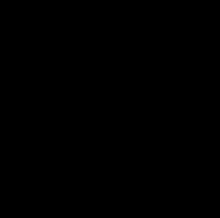Example: Consider f to be defined by{(0,0),(1,1),(2,2),(3,3),(1,2),(2,3),(3,1),(2,1),(3,2),(1,3)}This is a relation (not a function) since we can observe that 1 maps to 2 and 3, for instance. Limit of a function:Suppose f : R → R is defined on the real line and p,L ∈ R. Then we say that the limit of the function f is l ifFor every real ε > 0, there exists a real δ > 0 such that for all real x,0 < | x − p | < δ implies | f(x) − L | < ε.Mathematically it is represented as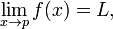Example: Find the limit of the function f(x) = (x2-6x + 8) / x-4, as x→5.Solution: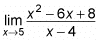The limit is 3, because f (5) = 3 and this function is continuous at x = 5.Such easy questions are not asked in the exam so it was just meant to clear the concept of limit. We now move on to a bit difficult question: Example: Find the limit of the function g(x) = √(x-4) -3 / (x-13) as x approaches 13.Solution: In such a question you first need to reduce the function in simple form so that the computation of limit becomes simple.First rationalize the numerator and denominator by multiplying by its conjugateSo, [√(x-4) -3] / (x-13) x [√(x-4) +3]/ [√(x-4) +3]On multiplying and solving we get the result,1/ √( x-4) +3Now since the terms have been simplified the limit can now be calculated by substituting the value of x as 13.Hence putting x=13 in the last equation we get the limit as 1/6. Watch this Video for more reference  Continuity of a function:A function y = f(x) is continuous at point x=a if the following three conditions are satisfied:(1)  f(a) is defined ,(2)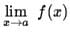exists (i.e., is finite) ,(3)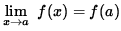A function is continuous when its graph is a single unbroken curve. This definition proves to be useful when it is possible to draw the graph of a function so that just by the graph the continuity of the function can be judged.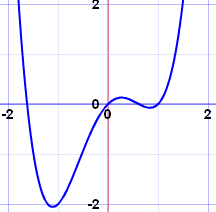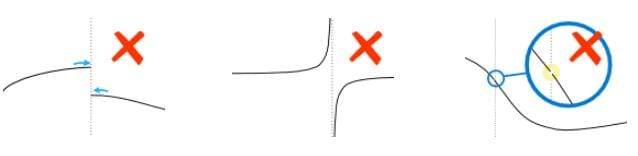Differentiation: Differentiation is an operation that allows us to find a function that outputs the rate of change of one variable with respect to another variable. The derivative of a function at a chosen input value describes the rate of change of the function near that input value. The process of finding a derivative is called differentiation. Geometrically, the derivative at a point is the slope of the tangent line to the graph of the function at that point, provided that the derivative exists and is defined at that point.Since Differential Calculus is new to the students as they do not study it in their 10th standard examination, so they are advised to master the topic by practicing questions on Limits, Continuity and Differentiability. The preparation of Differential Calculus also gives another opportunity to prepare and revise the chapter on Functions, Sets and Relations. To get an idea about the types of questions asked you may also consult the Previous Year Papers.`

The document Differential Calculus Notes - JEE is a part of JEE category.
All you need of JEE at this link: JEEUse Code STAYHOME200 and get INR 200 additional OFF

Track your progress, build streaks, highlight & save important lessons and more!

,

,

,

,

,

,

,

,

,

,

,

,

,

,

,

,

,

,

,

,

,

;